The OpenFOAM FoundationVector2D< Cmpt > Class Template Reference

Templated 2D Vector derived from VectorSpace adding construction from 2 components, element access using x() and y() member functions and the inner-product (dot-product). More...

Inheritance diagram for Vector2D< Cmpt >: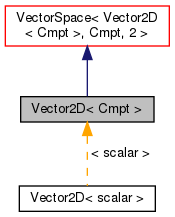[legend]
Collaboration diagram for Vector2D< Cmpt >: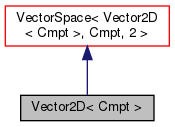[legend]

## Public Types

enum  components { X, Y }
Component labeling enumeration. More...

typedef Vector2D< labellabelType
Equivalent type of labels used for valid component indexing. More...Public Types inherited from VectorSpace< Vector2D< Cmpt >, Cmpt, 2 >
typedef VectorSpace< Vector2D< Cmpt >, Cmpt, Ncmpts > vsType
VectorSpace type. More...

typedef Cmpt cmptType
Component type. More...

## Public Member Functions

Vector2D ()
Construct null. More...

Vector2D (const Foam::zero)
Construct initialized to zero. More...

Vector2D (const VectorSpace< Vector2D< Cmpt >, Cmpt, 2 > &)
Construct given VectorSpace. More...

Vector2D (const Cmpt &vx, const Cmpt &vy)
Construct given two components. More...

Vector2D (Istream &)
Construct from Istream. More...

const Cmpt & x () const

const Cmpt & y () const

Cmpt & x ()

Cmpt & y ()

scalar perp (const Vector2D< Cmpt > &b) const
Perp dot product (dot product with perpendicular vector) More...Public Member Functions inherited from VectorSpace< Vector2D< Cmpt >, Cmpt, 2 >
VectorSpace ()
Construct null. More...

VectorSpace (const Foam::zero)
Construct initialized to zero. More...

VectorSpace (Istream &)
Construct from Istream. More...

VectorSpace (const VectorSpace< Form2, Cmpt2, Ncmpts > &)
Construct as copy of a VectorSpace with the same size. More...

const Cmpt & component (const direction) const

Cmpt & component (const direction)

void component (Cmpt &, const direction) const

void replace (const direction, const Cmpt &)

const ConstBlock< SubVector, BStart > block () const

const VectorSpace< Vector2D< Cmpt >, Cmpt, Ncmpts >::template ConstBlock< SubVector, BStart > block () const

const Cmpt & operator[] (const direction) const

Cmpt & operator[] (const direction)

void operator+= (const VectorSpace< Vector2D< Cmpt >, Cmpt, Ncmpts > &)

void operator-= (const VectorSpace< Vector2D< Cmpt >, Cmpt, Ncmpts > &)

void operator= (const Foam::zero)

void operator*= (const scalar)

void operator/= (const scalar)

## Static Public Attributes

static const direction rank = 1
Rank of Vector2D is 1. More...Static Public Attributes inherited from VectorSpace< Vector2D< Cmpt >, Cmpt, 2 >
static const direction dim
Dimensionality of space. More...

static const direction nComponents
Number of components in this vector space. More...

static const direction mRows

static const direction nCols

static const char *const typeName

static const char *const componentNames []

static const Vector2D< Cmpt > zero

static const Vector2D< Cmpt > one

static const Vector2D< Cmpt > max

static const Vector2D< Cmpt > min

static const Vector2D< Cmpt > rootMax

static const Vector2D< Cmpt > rootMinStatic Public Member Functions inherited from VectorSpace< Vector2D< Cmpt >, Cmpt, 2 >
static direction size ()
Return the number of elements in the VectorSpace = Ncmpts. More...

static Vector2D< Cmpt > uniform (const Cmpt &s)
Return a VectorSpace with all elements = s. More...Public Attributes inherited from VectorSpace< Vector2D< Cmpt >, Cmpt, 2 >
Cmpt v_ [Ncmpts]
The components of this vector space. More...

## Detailed Description

### template<class Cmpt> class Foam::Vector2D< Cmpt >

Templated 2D Vector derived from VectorSpace adding construction from 2 components, element access using x() and y() member functions and the inner-product (dot-product).

Source files

Definition at line 51 of file Vector2D.H.

## ◆ labelType

 typedef Vector2D

Equivalent type of labels used for valid component indexing.

Definition at line 59 of file Vector2D.H.

## ◆ components

 enum components

Component labeling enumeration.

Enumerator

Definition at line 69 of file Vector2D.H.

## ◆ Vector2D() [1/5]

 Vector2D ( )
inline

Construct null.

Definition at line 29 of file Vector2DI.H.

Referenced by Vector2D< scalar >::Vector2D().

Here is the caller graph for this function: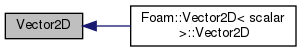## ◆ Vector2D() [2/5]

 Vector2D ( const Foam::zero )
inline

Construct initialized to zero.

Definition at line 34 of file Vector2DI.H.

## ◆ Vector2D() [3/5]

 Vector2D ( const VectorSpace< Vector2D< Cmpt >, Cmpt, 2 > & vs )
inline

Construct given VectorSpace.

Definition at line 42 of file Vector2DI.H.

## ◆ Vector2D() [4/5]

 Vector2D ( const Cmpt & vx, const Cmpt & vy )
inline

Construct given two components.

Definition at line 51 of file Vector2DI.H.

## ◆ Vector2D() [5/5]

 Vector2D ( Istream & is )
inline

Construct from Istream.

Definition at line 59 of file Vector2DI.H.

## ◆ x() [1/2]

 const Cmpt & x ( ) const
inline

Definition at line 68 of file Vector2DI.H.

Here is the caller graph for this function: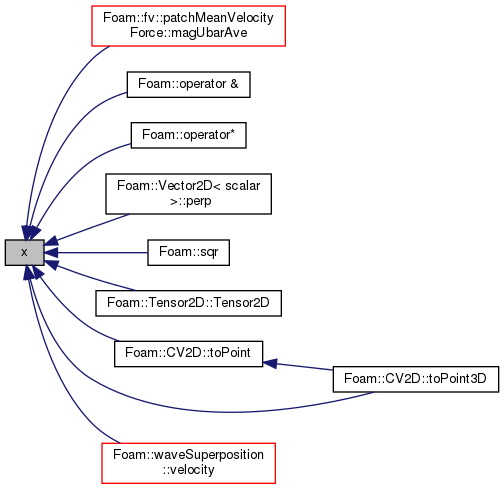## ◆ y() [1/2]

 const Cmpt & y ( ) const
inline

Definition at line 74 of file Vector2DI.H.

Here is the caller graph for this function: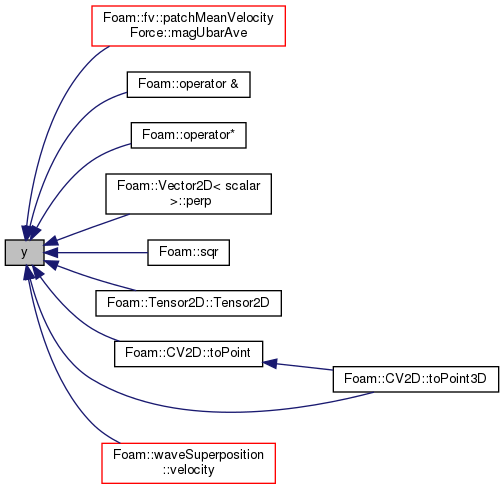## ◆ x() [2/2]

 Cmpt & x ( )
inline

Definition at line 81 of file Vector2DI.H.

## ◆ y() [2/2]

 Cmpt & y ( )
inline

Definition at line 87 of file Vector2DI.H.

## ◆ perp()

 scalar perp ( const Vector2D< Cmpt > & b ) const
inline

Perp dot product (dot product with perpendicular vector)

Definition at line 109 of file Vector2DI.H.

Referenced by line< Point, PointRef >::nearestDist().

Here is the caller graph for this function: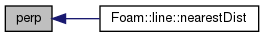## ◆ rank

 const direction rank = 1
static

Rank of Vector2D is 1.

Definition at line 65 of file Vector2D.H.

The documentation for this class was generated from the following files: